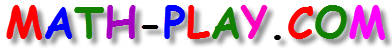#Adding Integers Game is a fun game that requires students to quickly identify whether the answer of an addition problem is positive, negative, or zero.

When playing this game, middle school students have to drag-and-drop twenty different addition problems into the correct boxes in less than half a minute. For each correct answer, students will earn a point, but each mistake will cost them a point. How many points can you earn? This game is a lot of fun and can be played on computers, iPads, and other tablets. You do not need to install an app to play this game on the iPad. Let the challange begin!

The game is based on the following Common Core Math Standards:

CCSS 6.NS.5
Understand that positive and negative numbers are used together to describe quantities having opposite directions or values (e.g., temperature above/below zero, elevation above/below sea level, credits/debits, positive/negative electric charge); use positive and negative numbers to represent quantities in real-world contexts, explaining the meaning of 0 in each situation.

CCSS 7.NS.1.b
Understand p + q as the number located a distance |q| from p, in the positive or negative direction depending on whether q is positive or negative. Show that a number and its opposite have a sum of 0 (are additive inverses).